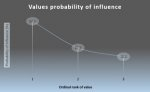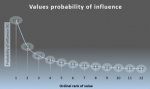# Please explain in mathematical terms what's happening here!?

#### Gareth1

##### New member
Hi Guys,

I'm not trained in mathematics, but I need to explain the following in such terms. Could you please assist?

• Three values are ranked ordinally (1 to 3) in terms of importance.
• Therefore: Value 1 > Value 2 > Value 3
• Each value carries the same weight. The sum of weights = 1. Therefore the individual weight is 1/3=0.33
• The ordinal importance is inversely proportional to the value's rank and is calculated as such: 1/(value weight*value rank) e.g. for value 1 which is the most important, the ordinal importance is: 1/(0.33*1)=3
• The sum of the ordinal importance values allows the relative importance (or the influence probability) to be calculated, which is essentially a percentage value. E.g. Value 1 influence probability is calculated: 3/(3+1.5+1)*100=54.5%

The table below summarises each value's parameters:

 Value Ordinal importance Influence Probability 1 3 54.5 2 1.5 27.3 3 1 18.2

If we plot the values graphically we have th
e following:What is already obvious is that we have a curve that is progressively flattening. Now if we extend the number of values to say 12, the progressive flattening of the curve is clearer.So here is my question: What is the nature of this function? I think it may be something like an inverse natural log (or whatever - I don't know).

I need to explain this in words so that if we didn't have the benefit of the images, that a mathematician would immediately understand what I am referring to.

Thank you maths gurus!

Gareth

#### Attachments

• 11.9 KB Views: 2

#### Jomo

##### Elite Member
Hi Guys,

I'm not trained in mathematics, but I need to explain the following in such terms. Could you please assist?

• Three values are ranked ordinally (1 to 3) in terms of importance.
• Therefore: Value 1 > Value 2 > Value 3
• Each value carries the same weight. The sum of weights = 1. Therefore the individual weight is 1/3=0.33
• The ordinal importance is inversely proportional to the value's rank and is calculated as such: 1/(value weight*value rank) e.g. for value 1 which is the most important, the ordinal importance is: 1/(0.33*1)=3
• The sum of the ordinal importance values allows the relative importance (or the influence probability) to be calculated, which is essentially a percentage value. E.g. Value 1 influence probability is calculated: 3/(3+1.5+1)*100=54.5%

The table below summarises each value's parameters:

 Value Ordinal importance Influence Probability 1 3 54.5 2 1.5 27.3 3 1 18.2

If we plot the values graphically we have th
e following:
View attachment 10691

What is already obvious is that we have a curve that is progressively flattening. Now if we extend the number of values to say 12, the progressive flattening of the curve is clearer.
View attachment 10693

So here is my question: What is the nature of this function? I think it may be something like an inverse natural log (or whatever - I don't know).

I need to explain this in words so that if we didn't have the benefit of the images, that a mathematician would immediately understand what I am referring to.

Thank you maths gurus!

Gareth
Gareth, I am not sure that you can find a sequence (or curve) that will contain all those 12+ points. It certainly doesn't seem to be an inverse log function. I'd start off with yn = k/n where k is a constant (like y = k/x)

Edit: I guess f(x) = 1/sqrt(quadratic eq) might be a better fit

Last edited: